# Java Program to Perform Addition, Subtraction, Multiplication and Division

In this tutorial you will learn about the Java Program to Perform Addition, Subtraction, Multiplication and Division and its application with practical example.

## Java Program to Perform Addition, Subtraction, Multiplication, and Division

In this tutorial, we will learn to create a Java program that will Addition, Subtraction, Multiplication, and Division using Java programming.

## Prerequisites

Before starting with this tutorial, we assume that you are the best aware of the following java programming topics:

• Operators in Java Programming.
• Basic Input and Output function in Java Programming.
• Basic Java programming.
• Arithmetic operations in Java Programming.

## Program to perform the arithmetic operations:-

In this program, we will first take two numbers in input from the user. Then will use the arithmetic operators to perform the operations on that numbers. At last, we will print the output to the user. Using the print function.

The operators are as follows:-

2. Subtraction Operator. “-“
3. Multiplication Operator. “*”
4. Divide Operator. “/”

## Output:-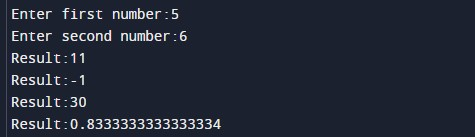In the above program, we have first initialized the required variable.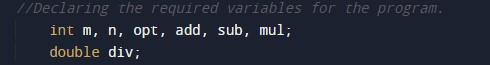• m = it will hold the integer value.
• n  = it will hold the integer value.

Input number from the user.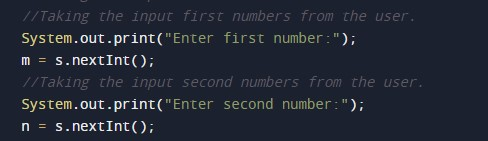Program Logic Code to perform the calculation.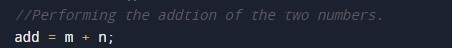Printing the addition of the program.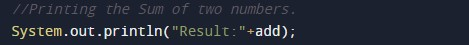In this tutorial we have learn about the Java Program to Perform Addition, Subtraction, Multiplication and Division and its application with practical example. I hope you will like this tutorial.